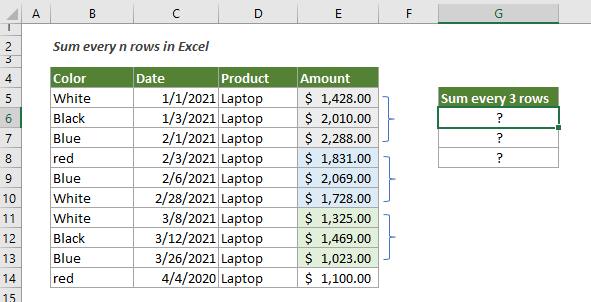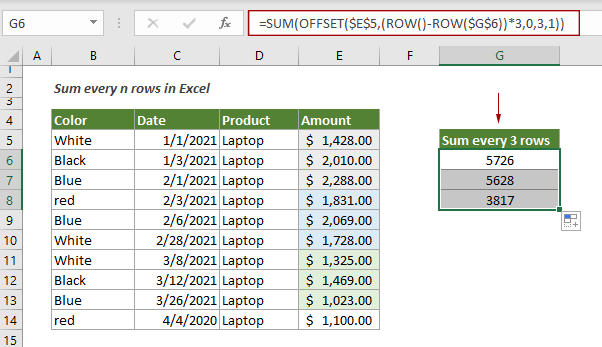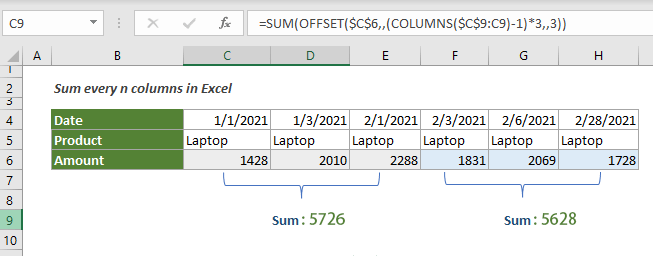## Sum every n rows or columns in a range in Excel

This tutorial explains two formulas based on the SUM and OFFSET functions to sum every n rows or columns in a specified range in Excel.

#### How to sum every n rows or columns in a given rang in Excel?

To sum every 3 rows in range E5:E14 as shown in the screenshot below, you can try the below formula.Generic Formulas

=SUM(OFFSET(A1,(ROW()-offset)*n,0,n,1))

Arguments

A1: Represents the first cell of the range you will sum;
N: It is a number that represents every n rows.

How to use this formula?

1. Select a blank cell to output the first result.

2. Copy or enter the below formula into it and press the Enter key. Select the result cell, drag its AutoFill Handle down to get the other results.

=SUM(OFFSET(\$E\$5,(ROW()-ROW(\$G\$6))*3,0,3,1))Notes:

1) In the formula, G6 is the cell where the formula sits.
2) To sum every n columns as shown on the screenshot below, please try this formula:

=SUM(OFFSET(\$C\$6,,(COLUMNS(\$C\$9:C9)-1)*3,,3))Explanation of the formula

=SUM(OFFSET(\$E\$5,(ROW()-ROW(\$G\$6))*3,0,3,1))

OFFSET(\$E\$5,(ROW()-ROW(\$G\$6))*3,0,3,1): Here use the OFFSET function to return a range of reference from the starting row of the return range. OFFSET(\$E\$5,0,0,3,1).
SUM(OFFSET(\$E\$5,0,0,3,1)): The number 3 indicates 3 rows need to be summed starting from E5, then the SUM function sums values in E5:E7 and returns 5726.

#### Related functions

Excel SUM function
The Excel SUM function adds values.

Excel OFFSET function
The Excel OFFSET function returns a reference to a cell or a range of cells that is offset from a specific cell by a given number of rows and columns.

Excel ROW function
The Excel ROW function returns the row number of a reference.

Excel COLUMNS function
The Excel COLUMNS function returns the total number of columns in a given array or reference.

#### Related formulas

Sum values by month (with or without year)
This tutorial demonstrates two formulas in detail to help summing values by certain month and year or summing values by certain month ignore year in Excel.

Sum values by the week of a given date
This tutorial talks about how to sum values by the week in which a given date falls

Sum values by weeknum or weekday
This tutorial demonstrates formulas step-by-step to help you sum values in a certain range based on the specified week number or weekday in Excel.

Sum every nth row or column in a range
This tutorial is going to show you how to create a formula based on the SUMPRODUCT, MOD and COLUMN functions to sum every nth row or column in a range in Excel.

### The Best Office Productivity Tools

#### Kutools for Excel - Helps You To Stand Out From Crowd

Would you like to complete your daily work quickly and perfectly? Kutools for Excel brings 300 powerful advanced features (Combine workbooks, sum by color, split cell contents, convert date, and so on...) and save 80% time for you.

• Designed for 1500 work scenarios, helps you solve 80% Excel problems.
• Reduce thousands of keyboard and mouse clicks every day, relieve your tired eyes and hands.
• Become an Excel expert in 3 minutes. No longer need to remember any painful formulas and VBA codes.
• 30-day unlimited free trial. 60-day money back guarantee. Free upgrade and support for 2 years.#### Office Tab - Enable Tabbed Reading and Editing in Microsoft Office (include Excel)

• One second to switch between dozens of open documents!
• Reduce hundreds of mouse clicks for you every day, say goodbye to mouse hand.
• Increases your productivity by 50% when viewing and editing multiple documents.
• Brings Efficient Tabs to Office (include Excel), Just Like Chrome, Firefox, And New Internet Explorer.